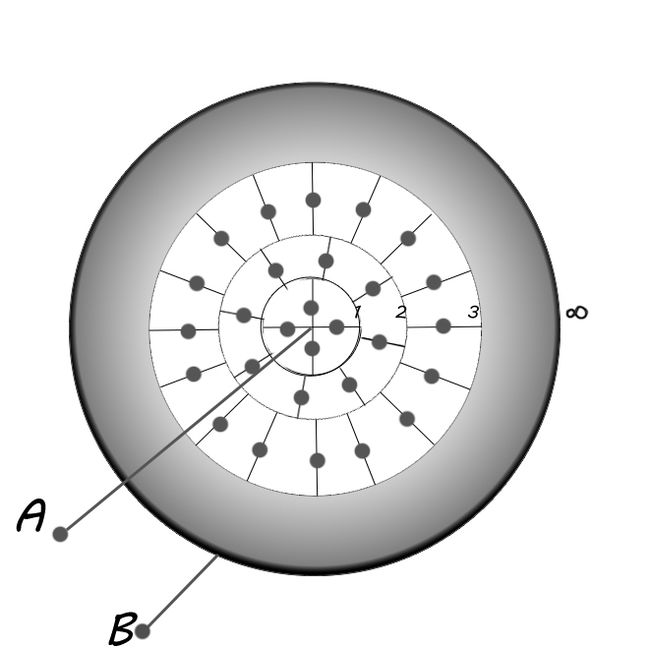# They are so manyIn the image above, gray dots are capacitors of capacitance $1\mu f$.

That gray area shows that, there is infinite arrangement of capacitors, such that first circle has 4 capacitors , second one has 8 capacitors, third 16 capacitors and next 32 and so on, as shown.

Find the equivalent capacitance in $\mu f$ between the two terminals $A$ (at the center) and $B$ (at infinity).

×